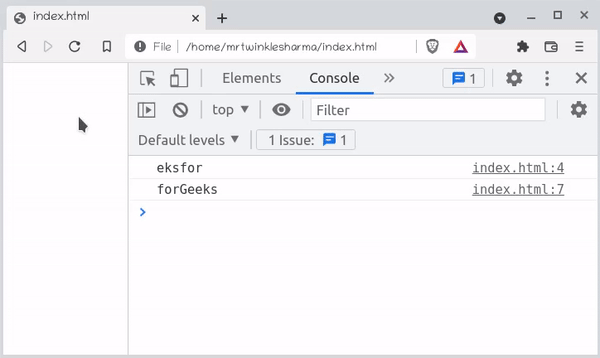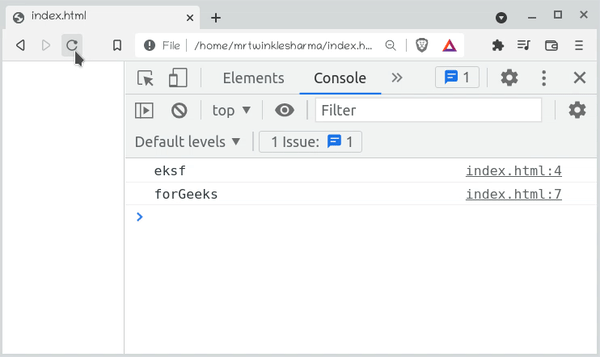# Which built-in method returns the characters in a string beginning at the specified location ?

• Last Updated : 16 Jan, 2022

In this article, we will know how to return the required sequence of characters in a string, beginning from a specified position, in Javascript. There are 2 built-in methods to accomplish this task:

• Using the substr() Method
• Using the slice() Method

We will explore these methods & will understand their implementation through the examples.

JavaScript substr() Method: The substr() Method built-in method that is used to return the characters in a string beginning at the specified location,

Syntax:

`str.substr(start, length);`

Parameters:

• start: It defines the index to start to extract the characters from the specified position and
• length: It defines the number of characters to be extracted starting from the start in the given string.

Return value: It returns a string that is the part of the given string. If the length is 0 or negative value then it returns an empty string.

Example: This example illustrates the concepts of the substr() method. In the first line, we have initialized a string, and called to substr() method with myString object. We have passed 2 as a starting index and 6 as the number of characters to be returned, then the ‘eksfor’ will be returned. If we have passed 5 as a starting index as we haven’t passed the second parameter, so, by default it will be considered till the end of the string and ‘forGeeks’ will be returned.

## HTML

 `<``script``>``    ``let myString = "GeeksforGeeks";``    ``let returnedStr1 = myString.substr(2, 6);``    ``console.log(returnedStr1);``    ``let returnedStr2 = myString.substr(5);``    ``console.log(returnedStr2);```

Output:Javascript substr() Method

JavaScript slice() Method: The slice() method is a built-in method that is used to create the slice of any string and then it returns the new string without affecting the original one.

Syntax:

`str.slice(beginIndex, endIndex);`

Parameter: The

• beginIndex: It is the beginning index of the slice, including the first index.
• endIndex: It is the last index where to stop slicing, excluding the last index.  By default, the endIndex is the string length.

Return: It returns a part or a slice of the given input string.

Example: This example illustrates the implementation of the slice() method. In the first line, we have initialized a string and called the slice() method by providing begin index as 2 and the last index is 6 so that it will return the characters from index 2 to 5 which are ‘eksf’. If we are calling the slice() method by providing the begin index as 5 and the end index will be taken as string length implicitly.

## HTML

 `<``script``>``    ``let myString = "GeeksforGeeks";``    ``let returnedStr1 = myString.slice(2, 6);``    ``console.log(returnedStr1);``    ``let returnedStr2 = myString.slice(5);``    ``console.log(returnedStr2);```

Output:Javascript slice() Method

My Personal Notes arrow_drop_up